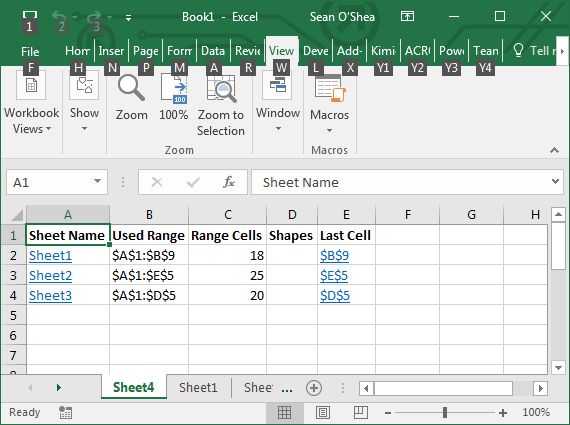Search

# Excel Macro to List Ranges on All Worksheets

If you've got a spreadsheet with a large number of worksheets, and you want to run a formula from one an all of them, but aren't sure of the respective data ranges on each, try this macro posted to the Contextures Blog of Debra Dalgleish.

So as we can see in this example we have three worksheets which each contain different ranges of data. Some of the ranges have blank cells within them.Entering Visual Basic (ALT + F11) and inserting the below code into a module with set up the macro. When you run it, a new worksheet will be created showing the full ranges on each worksheet.Sub ListSheetsRangeInfo() Dim ws As Worksheet Dim lCount As Long Dim wsTemp As Worksheet Dim rngF As Range Dim lFields As Long Dim strLC As String Dim strSh As String Dim sh As Shape Application.EnableEvents = False Application.ScreenUpdating = False On Error Resume Next Set wsTemp = Worksheets _ .Add(Before:=Sheets(1)) lCount = 2 lFields = 5 With wsTemp .Range(.Cells(1, 1), _ .Cells(1, lFields)).Value _ = Array( _ "Sheet Name", _ "Used Range", _ "Range Cells", _ "Shapes", _ "Last Cell") End With For Each ws In ActiveWorkbook.Worksheets If ws.Name <> wsTemp.Name Then strSh = "" strLC = ws.Cells _ .SpecialCells(xlCellTypeLastCell) _ .Address If ws.Shapes.Count > 0 Then For Each sh In ws.Shapes strSh = strSh & sh.TopLeftCell _ .Address & ", " Next sh strSh = Left(strSh, Len(strSh) - 2) End If With wsTemp .Range(.Cells(lCount, 1), _ .Cells(lCount, lFields)).Value _ = Array( _ ws.Name, _ ws.UsedRange.Address, _ ws.UsedRange.Cells.Count, _ strSh, _ strLC) 'add hyperlink to sheet name .Hyperlinks.Add _ Anchor:=.Cells(lCount, 1), _ Address:="", _ SubAddress:="'" & ws.Name _ & "'!A1", _ ScreenTip:=ws.Name, _ TextToDisplay:=ws.Name 'add hyperlink to last cell .Hyperlinks.Add _ Anchor:=.Cells(lCount, lFields), _ Address:="", _ SubAddress:="'" & ws.Name _ & "'!" & strLC, _ ScreenTip:=strLC, _ TextToDisplay:=strLC lCount = lCount + 1 End With End If Next ws With wsTemp .Range(.Cells(1, 1), .Cells(1, lFields)) _ .EntireColumn.AutoFit .Rows(1).Font.Bold = True End With

Application.EnableEvents = True Application.ScreenUpdating = True

End Sub